Ex 9.1

Chapter 9 Class 7 Perimeter and Area
Serial order wise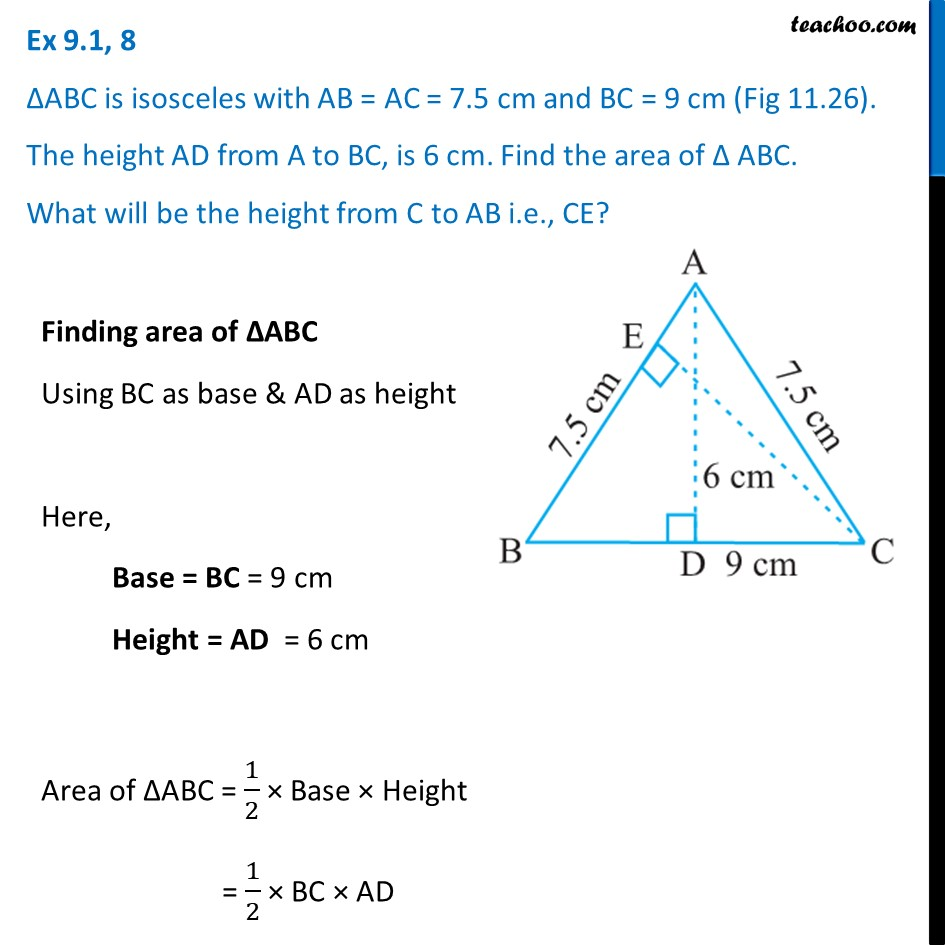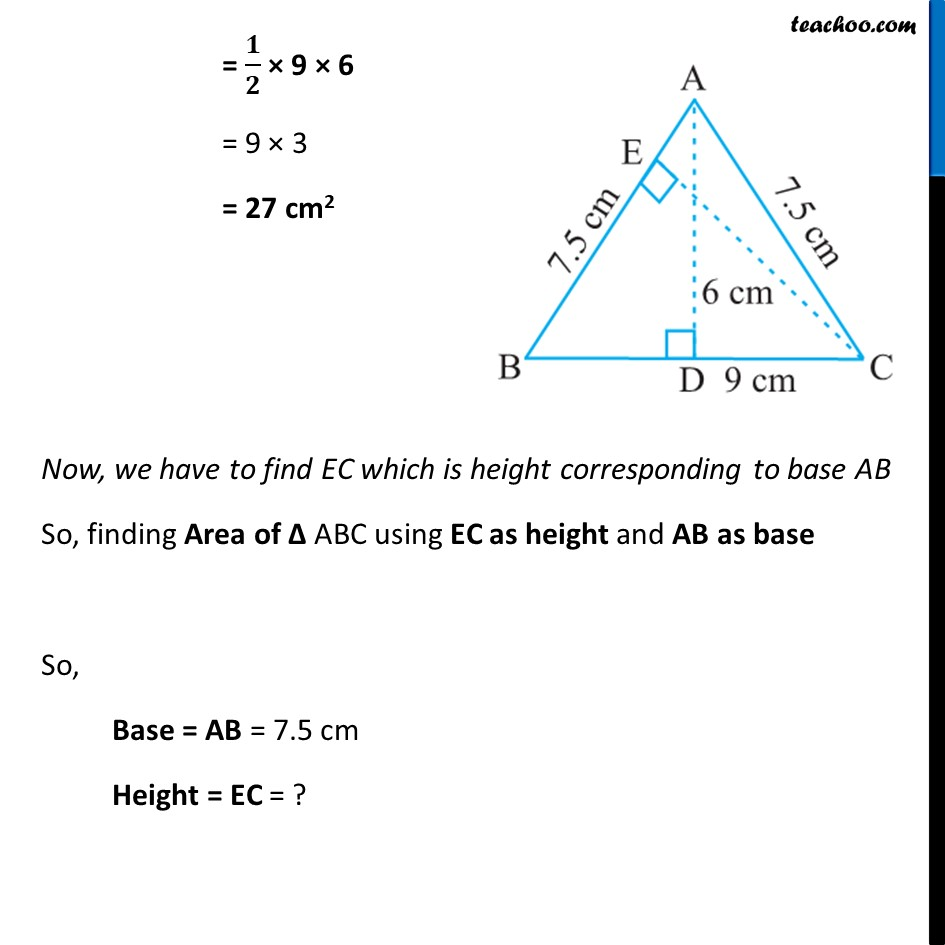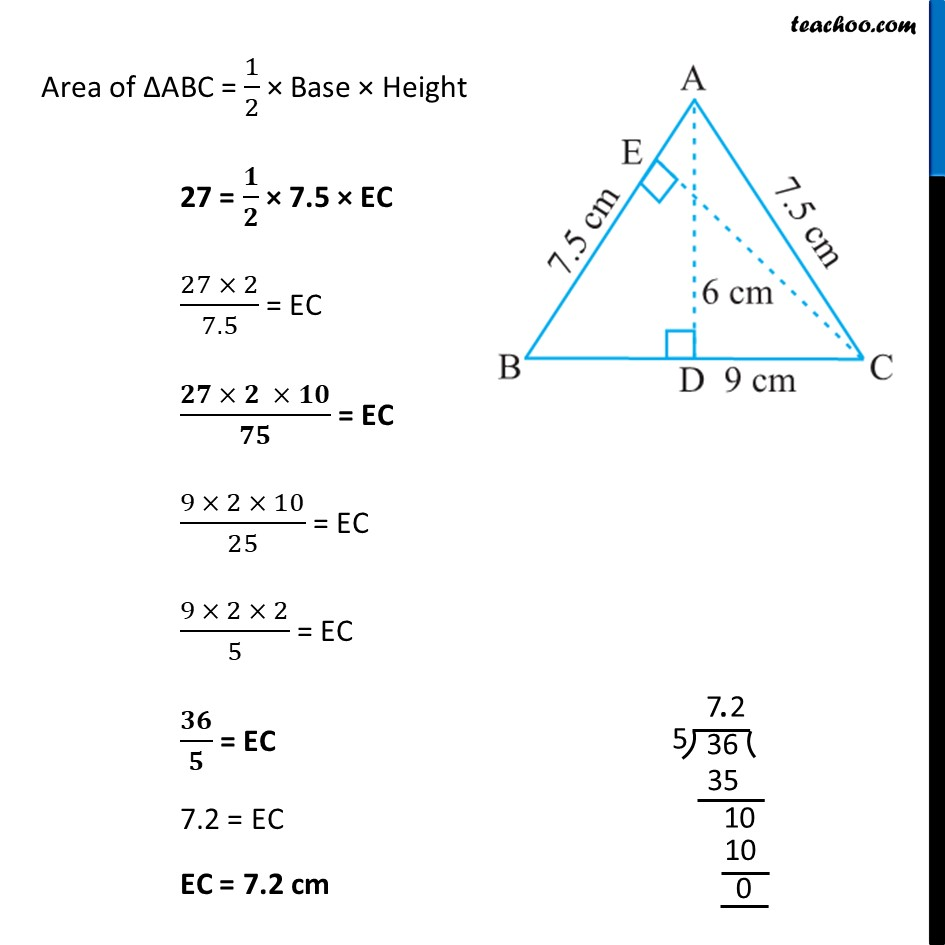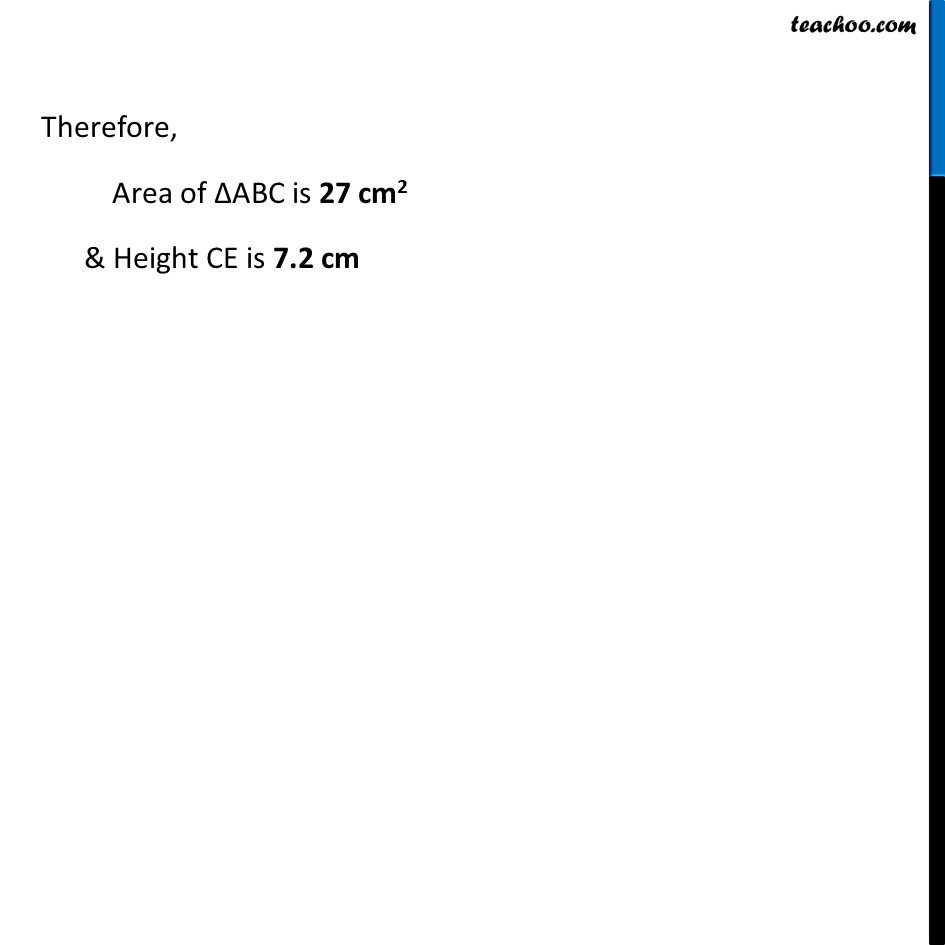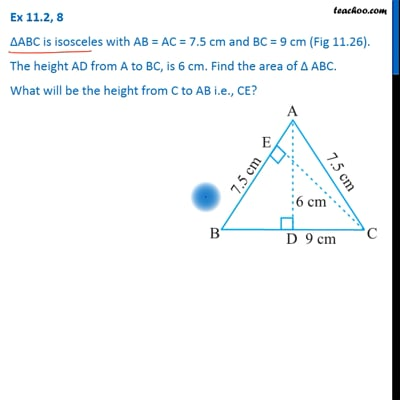This video is only available for Teachoo black users

Learn in your speed, with individual attention - Teachoo Maths 1-on-1 Class

### Transcript

Ex 9.1, 8 ΔABC is isosceles with AB = AC = 7.5 cm and BC = 9 cm (Fig 11.26). The height AD from A to BC, is 6 cm. Find the area of Δ ABC. What will be the height from C to AB i.e., CE? Finding area of ∆ABC Using BC as base & AD as height Here, Base = BC = 9 cm Height = AD = 6 cm Area of ∆ABC = 1/2 × Base × Height = 1/2 × BC × AD = 𝟏/𝟐 × 9 × 6 = 9 × 3 = 27 cm2 Now, we have to find EC which is height corresponding to base AB So, finding Area of Δ ABC using EC as height and AB as base So, Base = AB = 7.5 cm Height = EC = ? Area of ∆ABC = 1/2 × Base × Height 27 = 𝟏/𝟐 × 7.5 × EC (27 × 2)/7.5 = EC (𝟐𝟕 × 𝟐 × 𝟏𝟎)/𝟕𝟓 = EC (9 × 2 × 10)/25 = EC (9 × 2 × 2)/5 = EC 𝟑𝟔/𝟓 = EC 7.2 = EC EC = 7.2 cm Therefore, Area of ∆ABC is 27 cm2 & Height CE is 7.2 cm